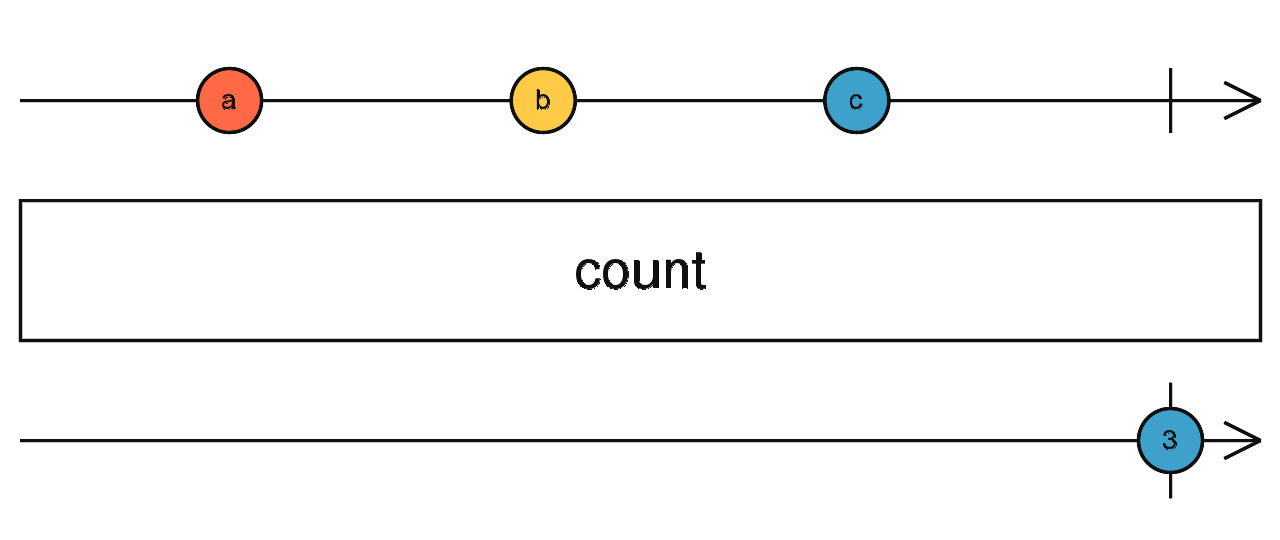/RxJS

# count

`function` `stable`

Counts the number of emissions on the source and emits that number when the source completes.

### `count<T>(predicate?: (value: T, index: number, source: Observable<T>) => boolean): OperatorFunction<T, number>`

#### Parameters

 predicate Optional. Default is `undefined`. A boolean function to select what values are to be counted. It is provided with arguments of: `value`: the value from the source Observable. `index`: the (zero-based) "index" of the value from the source Observable. `source`: the source Observable instance itself.

#### Returns

`OperatorFunction<T, number>`: An Observable of one number that represents the count as described above.

## Description

Tells how many values were emitted, when the source completes.`count` transforms an Observable that emits values into an Observable that emits a single value that represents the number of values emitted by the source Observable. If the source Observable terminates with an error, `count` will pass this error notification along without emitting a value first. If the source Observable does not terminate at all, `count` will neither emit a value nor terminate. This operator takes an optional `predicate` function as argument, in which case the output emission will represent the number of source values that matched `true` with the `predicate`.

## Examples

Counts how many seconds have passed before the first click happened

```import { fromEvent, interval } from 'rxjs';
import { count, takeUntil } from 'rxjs/operators';

const seconds = interval(1000);
const clicks = fromEvent(document, 'click');
const secondsBeforeClick = seconds.pipe(takeUntil(clicks));
const result = secondsBeforeClick.pipe(count());
result.subscribe(x => console.log(x));```

Counts how many odd numbers are there between 1 and 7

```import { range } from 'rxjs';
import { count } from 'rxjs/operators';

const numbers = range(1, 7);
const result = numbers.pipe(count(i => i % 2 === 1));
result.subscribe(x => console.log(x));
// Results in:
// 4```